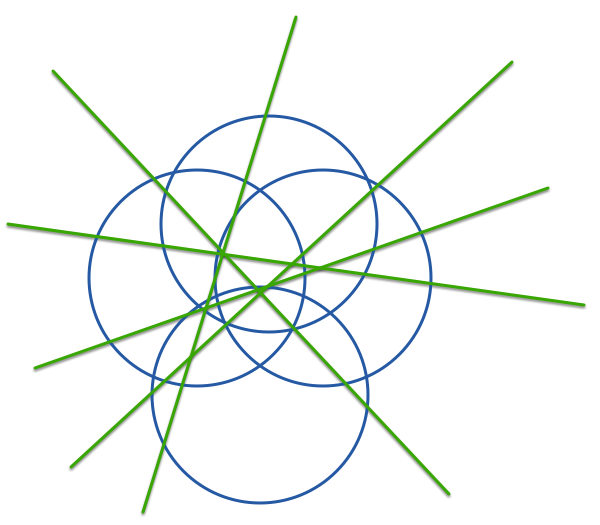# Intersecting Lines And CirclesThe maximum number of points of intersection of 5 straight lines and 4 circles in a plane is $\text{\_\_\_\_\_\_\_\_\_\_}$.

×